This resource function is obsolete. Use the paclet Wolfram/QuantumFramework instead.

Function Repository Resource:

# QuantumSpectralDecomposition

Perform a spectral decomposition (diagonalization) on a quantum state or operator

Contributed by: Jonathan Gorard and Ruhi Shah
 ResourceFunction["QuantumSpectralDecomposition"][QuantumDiscreteState[…]] performs a spectral decomposition on the specified QuantumDiscreteState. ResourceFunction["QuantumSpectralDecomposition"][QuantumDiscreteOperator[…]] performs a spectral decomposition on the specified QuantumDiscreteOperator. ResourceFunction["QuantumSpectralDecomposition"][QuantumMeasurementOperator[…]] performs a spectral decomposition on the specified QuantumMeasurementOperator. ResourceFunction["QuantumSpectralDecomposition"][QuantumHamiltonianOperator[…]] performs a spectral decomposition on the specified QuantumHamiltonianOperator. ResourceFunction["QuantumSpectralDecomposition"][QuantumCircuitOperator[…]] performs a spectral decomposition on the specified QuantumCircuitOperator, represented as a QuantumDiscreteOperator.

## Details

The spectral decomposition in linear algebra and functional analysis represents a Hermitian/normal matrix (or linear operator) as a diagonal matrix/operator in some basis. In the context of quantum information theory, it diagonalizes a density matrix, evolution operator, measurement operator, Hamiltonian operator or circuit operator by introducing an appropriate orthonormal basis.
ResourceFunction["QuantumSpectralDecomposition"] will return a QuantumDiscreteState object if the input was a QuantumDiscreteState object, a QuantumDiscreteOperator object if the input was a QuantumDiscreteOperator object, etc. The only exception to this rule is the QuantumCircuitOperator object, whose spectral decomposition is always a QuantumDiscreteOperator object (i.e. ResourceFunction["QuantumSpectralDecomposition"] always returns the operator representation of the resulting circuit).
ResourceFunction["QuantumSpectralDecomposition"] is defined for projection-valued QuantumMeasurementOperator objects, but not for positive operator-valued QuantumMeasurementOperator objects.
The basis elements in the resulting orthonormal basis are named s1,s2,.

## Examples

### Basic Examples (5)

Create a two-qubit pure discrete quantum state in the computational basis (default):

 In:=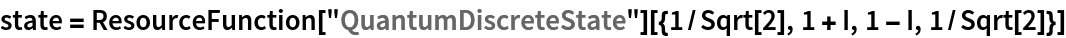Out=In:=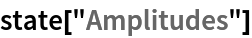Out=In:=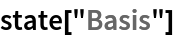Out=Perform a spectral decomposition of the state, resulting in a pure state with a diagonalized density matrix and a new (spectral-decomposed) basis:

 In:=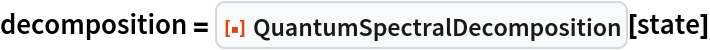Out=In:=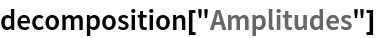Out=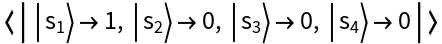In:=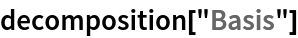Out=Show the basis elements of the new (spectral-decomposed) basis:

 In:=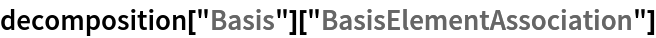Out=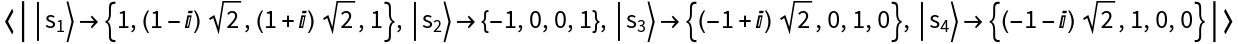Perform a spectral decomposition of a single-qubit mixed discrete quantum state in the computational basis instead, resulting in a mixed state with a diagonalized density matrix:

 In:=Out=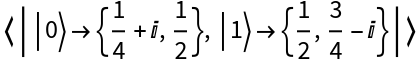In:=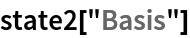Out=In:=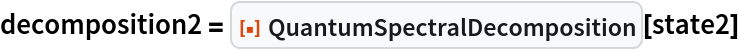Out=In:=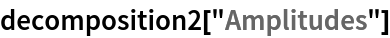Out=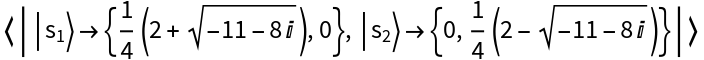Show the new (spectral-decomposed) basis:

 In:=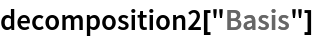Out=In:=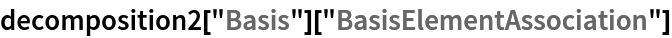Out=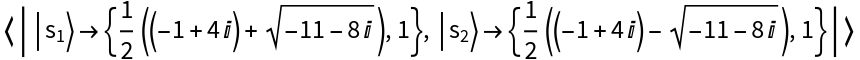Perform a spectral decomposition of an arity-2 QuantumDiscreteOperator object, resulting in a QuantumDiscreteOperator object with a diagonalized matrix representation:

 In:=Out=Show the new (spectral-decomposed) basis:

 In:=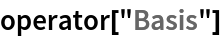Out=In:=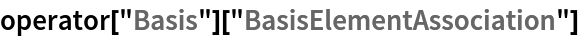Out=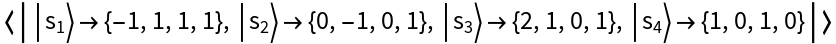Perform a spectral decomposition of an arity-3 projection-valued QuantumMeasurementOperator object, resulting in a projection-valued QuantumMeasurementOperator object with a diagonalized matrix representation:

 In:=Out=Show the new (spectral decomposed) basis:

 In:=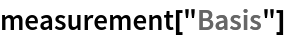Out=In:=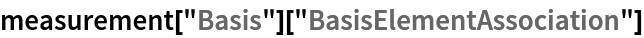Out=Perform a spectral decomposition on an arity-1 QuantumHamiltonianOperator object, resulting in a QuantumHamiltonianOperator object with a diagonalized matrix representation:

 In:=Out=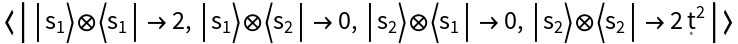Show the new (spectral-decomposed) basis:

 In:=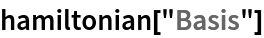Out=In:=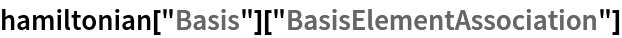Out=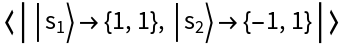Perform a spectral decomposition of an arity-2 QuantumCircuitOperator object, resulting in a QuantumDiscreteOperator object with a diagonalized matrix representation:

 In:=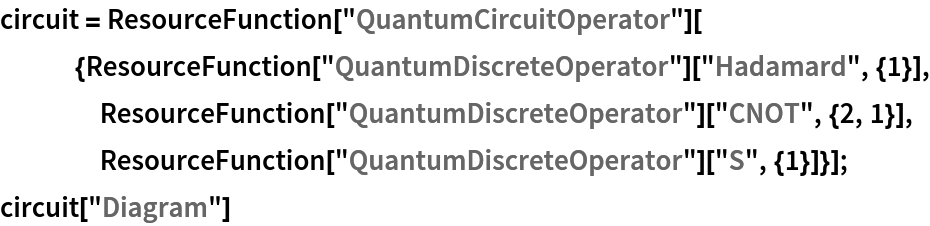Out=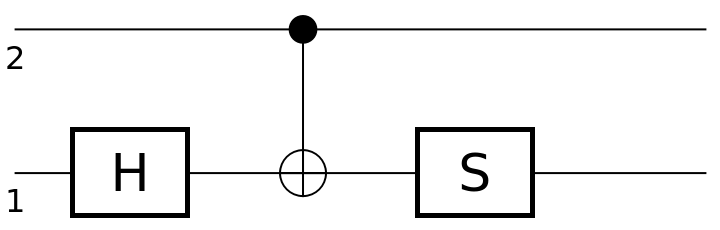In:=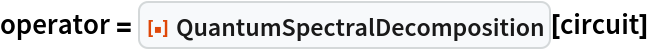Out=Show the resulting operator association:

 In:=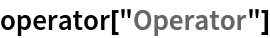Out=Show the new (spectral-decomposed) basis:

 In:=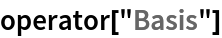Out=In:=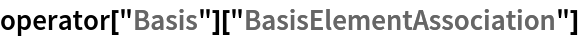Out=### Scope (2)

QuantumSpectralDecomposition can perform spectral decompositions of quantum objects in arbitrary bases:

 In:=Out=In:=Out=In:=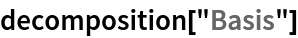Out=Perform spectral decompositions of higher-dimensional quantum objects:

 In:=Out=In:=Out=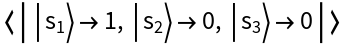In:=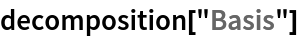Out=Jonathan Gorard

## Version History

• 1.0.0 – 02 June 2021